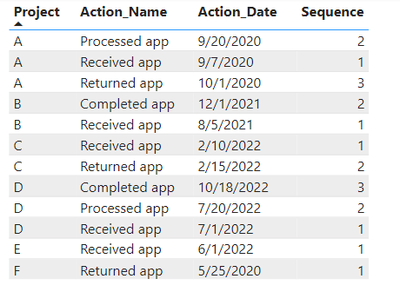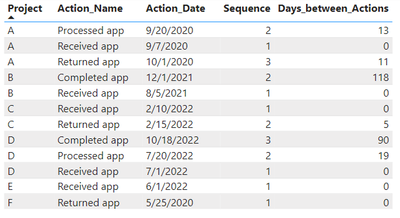cancel
Showing results for
Did you mean:Helper I

## Filter Multiple Criteria

Hi, all. I have the following table with multiple projects.I need a new column called Fiscal Year that is calculated based on the following criteria:

1. Must be the MAX Sequence for the project
2. Must be an an Action_Name of either “Returned app” or “Completed app”
• If the two criteria above apply and the Action_Date is 10/01/2020 – 09/30/2021, then Fiscal Year = “FY21”
• If the first two criteria apply and the Action_Date is 10/01/2021 – 09/30/2022, then Fiscal Year = “FY22”
• If the first two criteria apply and the Action_Date is 10/01/2022 – 09/30/2023, then Fiscal Year = “FY23”
• If nothing applies, then BLANK
1 ACCEPTED SOLUTIONSuper User

@PBInewbie17 Try:

``````FY Column =
VAR __Seq = [Sequence]
VAR __Project = [Project]
VAR __Action = [Action_Name]
VAR __Table = FILTER('Table',[Project] = __Project)
VAR __MaxSeq = MAXX(__Table,[Sequence])
RETURN
IF(
__Seq = __MaxSeq && ( __Action = "Returned app" || __Action = "Completed app" ),
SWITCH(TRUE(),
[Action_Date] >= DATE(2020,10,1) && [Action_Date] <= DATE(2021,9,30),"FY21",
[Action_Date] >= DATE(2021,10,1) && [Action_Date] <= DATE(2022,9,30),"FY21",
[Action_Date] >= DATE(2022,10,1) && [Action_Date] <= DATE(2023,9,30),"FY21",
BLANK()
),
BLANK()
)``````

Become an expert!: Enterprise DNA
External Tools: MSHGQM
Latest book!:
Mastering Power BI 2nd EditionDAX is easy, CALCULATE makes DAX hard...
2 REPLIES 2Super User

@PBInewbie17 Try:

``````FY Column =
VAR __Seq = [Sequence]
VAR __Project = [Project]
VAR __Action = [Action_Name]
VAR __Table = FILTER('Table',[Project] = __Project)
VAR __MaxSeq = MAXX(__Table,[Sequence])
RETURN
IF(
__Seq = __MaxSeq && ( __Action = "Returned app" || __Action = "Completed app" ),
SWITCH(TRUE(),
[Action_Date] >= DATE(2020,10,1) && [Action_Date] <= DATE(2021,9,30),"FY21",
[Action_Date] >= DATE(2021,10,1) && [Action_Date] <= DATE(2022,9,30),"FY21",
[Action_Date] >= DATE(2022,10,1) && [Action_Date] <= DATE(2023,9,30),"FY21",
BLANK()
),
BLANK()
)``````

Become an expert!: Enterprise DNA
External Tools: MSHGQM
Latest book!:
Mastering Power BI 2nd EditionDAX is easy, CALCULATE makes DAX hard...Helper I

Thank you, Greg. One other caveat: I have another column called Days_between_Actions that is used to calculate the total number of days to complete a project (e.g., Project A took 24 days to complete [13 + 11 = 24]). The formula below is excluding certain days from the calculation. For example, it's showing Project A as 11 days instead of the correct 24. How can I adjust the formula to ensure the Days_between_Actions column isn't affected?FY Column =
VAR __Seq = [Sequence]
VAR __Project = [Project]
VAR __Action = [Action_Name]
VAR __Table =
FILTER ( 'Table', [Project] = __Project )
VAR __MaxSeq =
MAXX ( __Table, [Sequence] )
RETURN
IF (
__Seq = __MaxSeq
&& ( __Action = "Returned app"
|| __Action = "Completed app" ),
SWITCH (
TRUE (),
[Action_Date] >= DATE ( 2020, 10, 1 )
&& [Action_Date] <= DATE ( 2021, 9, 30 ), "FY21",
[Action_Date] >= DATE ( 2021, 10, 1 )
&& [Action_Date] <= DATE ( 2022, 9, 30 ), "FY22",
[Action_Date] >= DATE ( 2022, 10, 1 )
&& [Action_Date] <= DATE ( 2023, 9, 30 ), "FY23",
BLANK ()
),
BLANK ()
)